In networking,

• It is a unique address assigned to each computing device in an IP network.
• ISP assigns IP Address to all the devices present on its network.
• Computing devices use IP Address to identify and communicate with other devices in the IP network.

IP Addresses may be of the following two types-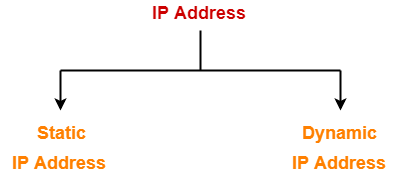• Static IP Address is an IP Address that once assigned to a network element always remains the same.
• They are configured manually.

### NOTE

• Some ISPs do not provide static IP addresses.

• Dynamic IP Address is a temporarily assigned IP Address to a network element.
• It can be assigned to a different device if it is not in use.
• DHCP or PPPoE assigns dynamic IP addresses.

• IP Address is a 32 bit binary address written as 4 numbers separated by dots.
• The 4 numbers are called as octets where each octet has 8 bits.
• The octets are divided into 2 components- Net ID and Host ID.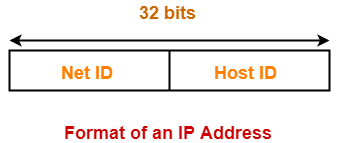1. Network ID represents the IP Address of the network and is used to identify the network.
2. Host ID represents the IP Address of the host and is used to identify the host within the network.

Example of an IP Address is-

00000001.10100000.00001010.11110000

(Binary Representation)

OR

1.160.10.240

(Decimal Representation)

There are two systems in which IP Addresses are classified-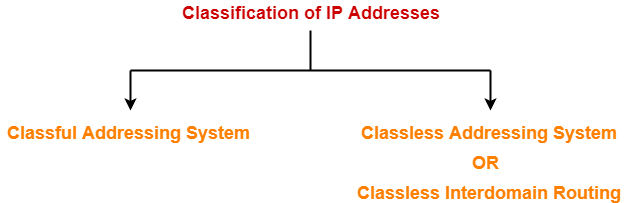In Classful Addressing System, IP Addresses are organized into following 5 classes-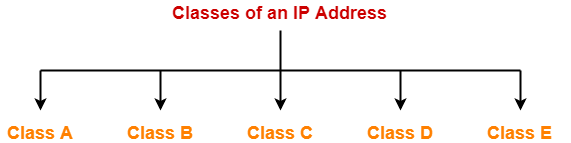1. Class A
2. Class B
3. Class C
4. Class D
5. Class E

## 1. Class A-

 If the 32 bit binary address starts with a bit 0, then IP Address belongs to class A.

• The first 8 bits are used for the Network ID.
• The remaining 24 bits are used for the Host ID.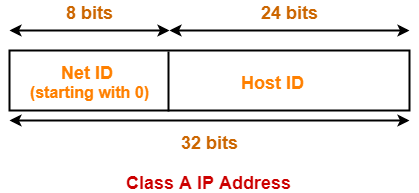### Total Number Of IP Addresses-

Total number of IP Addresses available in class A

= Numbers possible due to remaining available 31 bits

= 231

### Total Number Of Networks-

Total number of networks available in class A

= Numbers possible due to remaining available 7 bits in the Net ID – 2

= 27 – 2

= 126

(The reason of subtracting 2 is explained later.)

### Total Number Of Hosts-

Total number of hosts that can be configured in class A

= Numbers possible due to available 24 bits in the Host ID – 2

= 224 – 2

(The reason of subtracting 2 is explained later.)

### Range Of 1st Octet-

We have-

• Minimum value of 1st octet = 00000000 = 0
• Maximum value of 1st octet = 01111111 = 127

From here,

• Range of 1st octet = [0, 127]
• But 2 networks are reserved and unused.
• So, Range of 1st octet = [1, 126]

## Use-

• Class A is used by organizations requiring very large size networks like NASA, Pentagon etc.

## 2. Class B-

 If the 32 bit binary address starts with bits 10, then IP Address belongs to class B.

• The first 16 bits are used for the Network ID.
• The remaining 16 bits are used for the Host ID.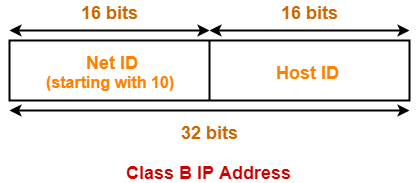### Total Number Of IP Addresses-

Total number of IP Addresses available in class B

= Numbers possible due to remaining available 30 bits

= 230

### Total Number Of Networks-

Total number of networks available in class B

= Numbers possible due to remaining available 14 bits in the Net ID

= 214

### Total Number Of Hosts-

Total number of hosts that can be configured in class B

= Numbers possible due to available 16 bits in the Host ID – 2

= 216 – 2

### Range Of 1st Octet-

We have-

• Minimum value of 1st octet = 10000000 = 128
• Maximum value of 1st octet = 10111111 = 191

So, Range of 1st octet = [128, 191]

## Use-

• Class B is used by organizations requiring medium size networks like IRCTC, banks etc.

## 3. Class C-

 If the 32 bit binary address starts with bits 110, then IP Address belongs to class C.

• The first 24 bits are used for the Network ID.
• The remaining 8 bits are used for the Host ID.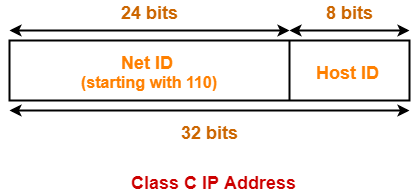### Total Number Of IP Addresses-

Total number of IP Addresses available in class C

= Numbers possible due to remaining available 29 bits

= 229

### Total Number Of Networks-

Total number of networks available in class C

= Numbers possible due to remaining available 21 bits in the Net ID

= 221

### Total Number Of Hosts-

Total number of hosts that can be configured in class C

= Numbers possible due to available 8 bits in the Host ID – 2

= 28 – 2

### Range Of 1st Octet-

We have-

• Minimum value of 1st octet = 11000000 = 192
• Maximum value of 1st octet = 110111111 = 223

So, Range of 1st octet = [192, 223]

## Use-

• Class C is used by organizations requiring small to medium size networks.
• For example- engineering colleges, small universities, small offices etc.

## 4. Class D-

 If the 32 bit binary address starts with bits 1110, then IP Address belongs to class D.

• Class D is not divided into Network ID and Host ID.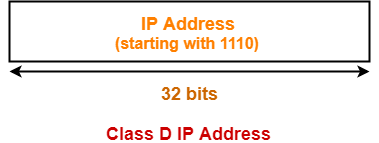### Total Number Of IP Addresses-

Total number of IP Addresses available in class D

= Numbers possible due to remaining available 28 bits

= 228

### Range Of 1st Octet-

We have-

• Minimum value of 1st octet = 11100000 = 224
• Maximum value of 1st octet = 11101111 = 239

So, Range of 1st octet = [224, 239]

## Use-

• Class D is reserved for multicasting.
• In multicasting, there is no need to extract host address from the IP Address.
• This is because data is not destined for a particular host.

## 5. Class E-

 If the 32 bit binary address starts with bits 1111, then IP Address belongs to class E.

• Class E is not divided into Network ID and Host ID.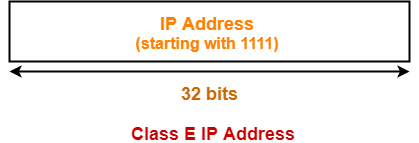### Total Number Of IP Addresses-

Total number of IP Addresses available in class E

= Numbers possible due to remaining available 28 bits

= 228

### Range Of 1st Octet-

We have-

• Minimum value of 1st octet = 11110000 = 240
• Maximum value of 1st octet = 11111111 = 255

So, Range of 1st octet = [240, 255]

## Use-

• Class E is reserved for future or experimental purposes.

All the classes of IP Address are summarized in the following table-

 Class of IP Address Total Number of IP Addresses 1st Octet Decimal Range Number of Networks available Hosts per network Default Subnet Mask Class A 231 1 – 126 27 – 2 224 – 2 255.0.0.0 Class B 230 128 – 191 214 216 – 2 255.255.0.0 Class C 229 192 – 223 221 28 – 2 255.255.255.0 Class D 228 224 – 239 Not defined Not defined Not defined Class E 228 240 – 254 Not defined Not defined Not defined

## Note-01:

• All the hosts in a single network always have the same network ID but different Host ID.
• However, two hosts in two different networks can have the same host ID.

## Note-02:

• A single network interface can be associated with more than one IP Address.

## Note-03:

• There is no relation between MAC Address and IP Address of a host.

## Note-04:

• IP Address of the network called Net ID is obtained by setting all the bits for Host ID to zero.

## Note-05:

• Class A Networks accounts for half of the total available IP Addresses.

## Note-06:

 In class A, total number of IP Addresses available for networks are 2 less.

• This is to account for the two reserved network IP Addresses 0.xxx.xxx.xxx and 127.xxx.xxx.xxx.
• IP Address 127.0.0.1 is reserved for loopback address used for software testing.

## Note-07:

 In all the classes, total number of hosts that can be configured are 2 less.

• This is to account for the two reserved IP addresses in which all the bits for host ID are either zero or one.
• When all Host ID bits are 0, it represents the Network ID for the network.
• When all Host ID bits are 1, it represents the Broadcast Address.

## Note-08:

• Only those devices which have the network layer will have IP Address.
•  So, switches, hubs and repeaters does not have any IP Address.

Watch this Video Lecture

Next Article- Casting | Types of Casting

Get more notes and other study material of Computer Networks.

Watch video lectures by visiting our YouTube channel LearnVidFun.

SummaryArticle Name